Next: JFET Common Source Amplifier Up: The Junction Field Effect Previous: Principles of Operation

## Small-Signal AC Model

The JFET characteristic curves can be described by an equation of the form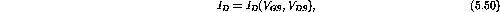where the function varies with the particular transistor. This expression yields the AC relationship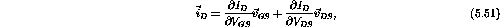where the AC currents and voltages are complex but the partial derivatives evaluate to real numbers. In the pinch-off region the curves of constant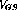are essential flat (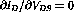) and allow the equation to be written as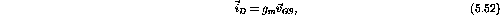where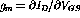is the transconductance.

Doug Gingrich
Tue Jul 13 16:55:15 EDT 1999